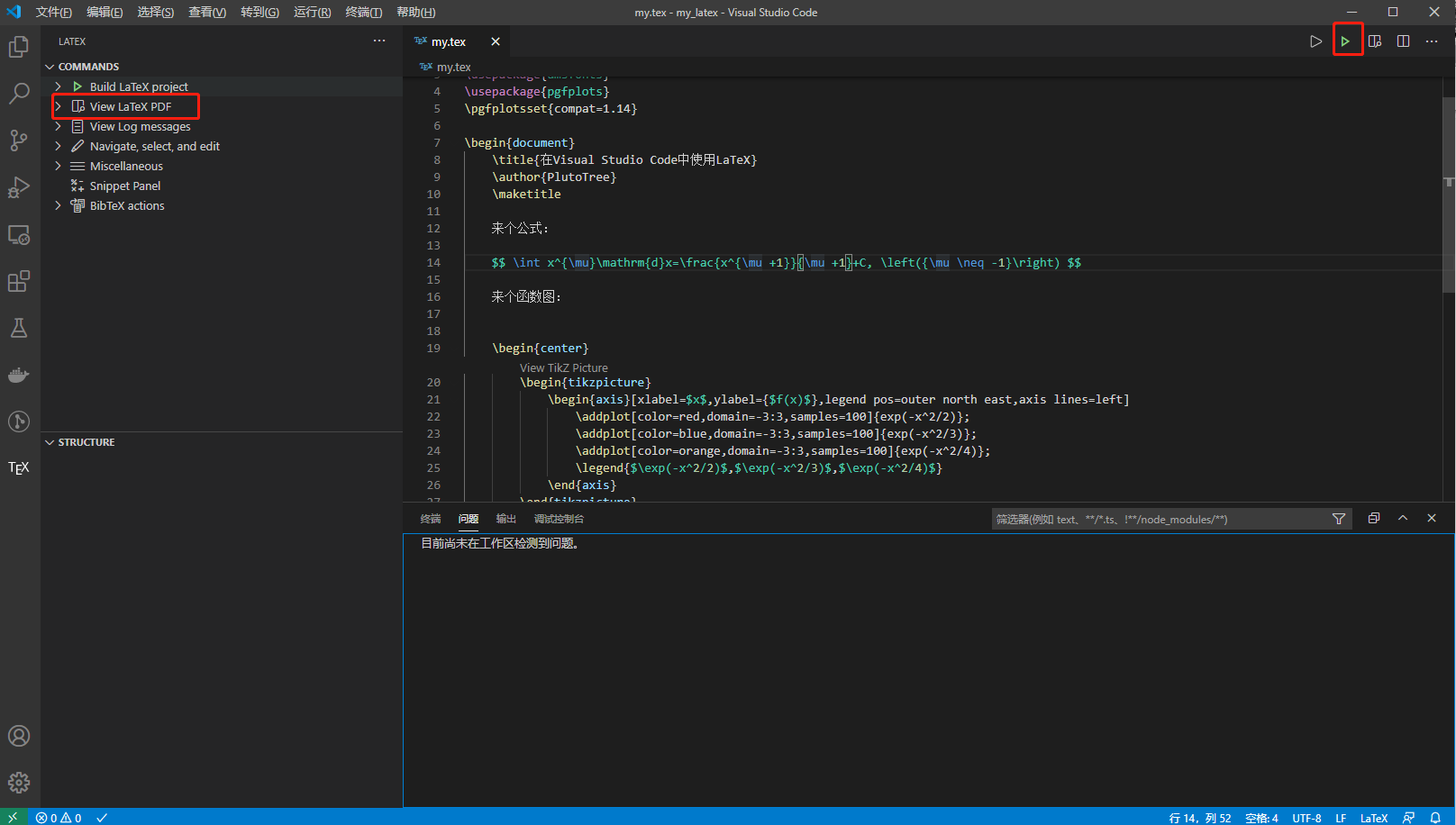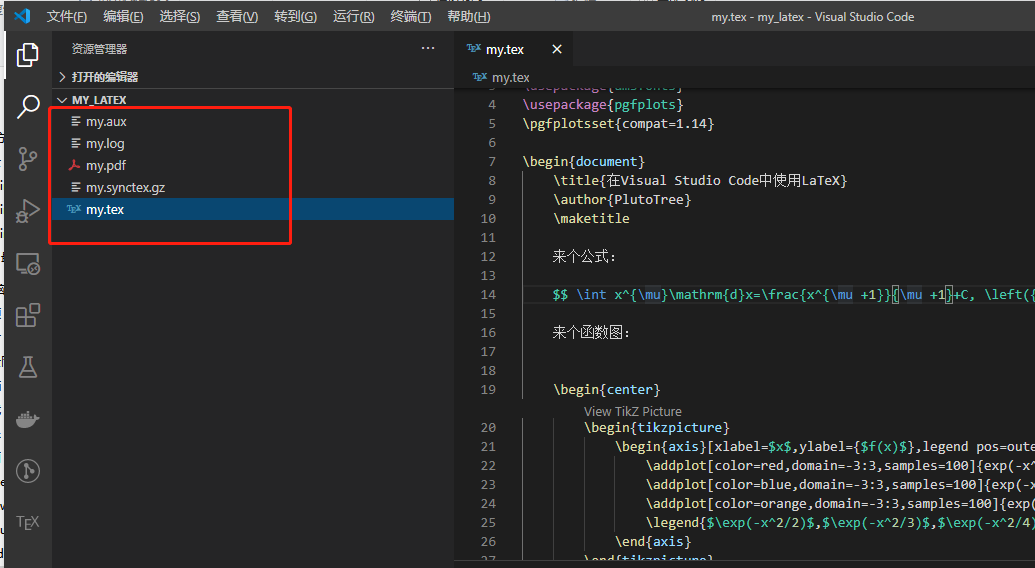## 关于LaTex

LaTeX是一种基于TeX的排版系统，而TeX是高德纳在发表《计算机程序艺术》的时候，因为当时的排版软件无法达到他的要求，自己编写的（牛人就是这么牛）。LaTeX非常适用于生成高质量的科技文章，在学术界用的较多，期刊杂志都会提供LaTex的模板，对于工科研究生来说算是必备的技能了。LaTeX作为一种标记语言，遵循的一个基本理念就是呈现和内容分离，和现在的markdown有点类似。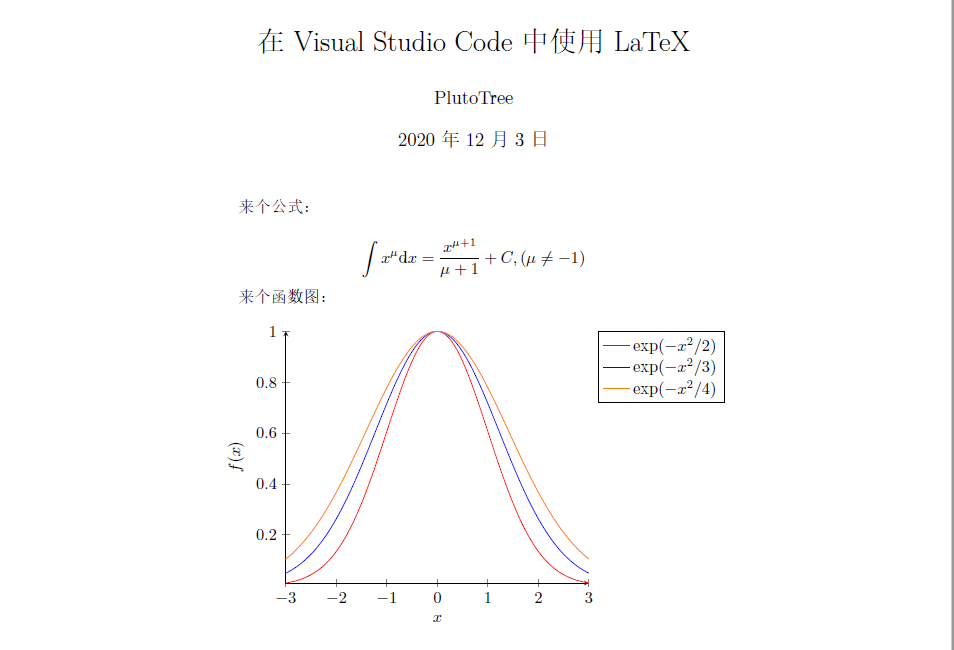\documentclass{article}
\usepackage[UTF8]{ctex}
\usepackage{amsfonts}
\usepackage{pgfplots}
\pgfplotsset{compat=1.14}

\begin{document}
\title{在Visual Studio Code中使用LaTeX}
\author{PlutoTree}
\maketitle

来个公式：

$$\int x^{\mu}\mathrm{d}x=\frac{x^{\mu +1}}{\mu +1}+C, \left({\mu \neq -1}\right)$$

来个函数图：

\begin{center}
\begin{tikzpicture}
\begin{axis}[xlabel=$x$,ylabel={$f(x)$},legend pos=outer north east,axis lines=left]
\legend{$\exp(-x^2/2)$,$\exp(-x^2/3)$,$\exp(-x^2/4)$}
\end{axis}
\end{tikzpicture}
\end{center}
\end{document}


LaTeX感兴趣的话，可以参考相关资料，这里还是先回归正题，有空的话我也会写写LaTeX的使用文章。

## 安装TexLive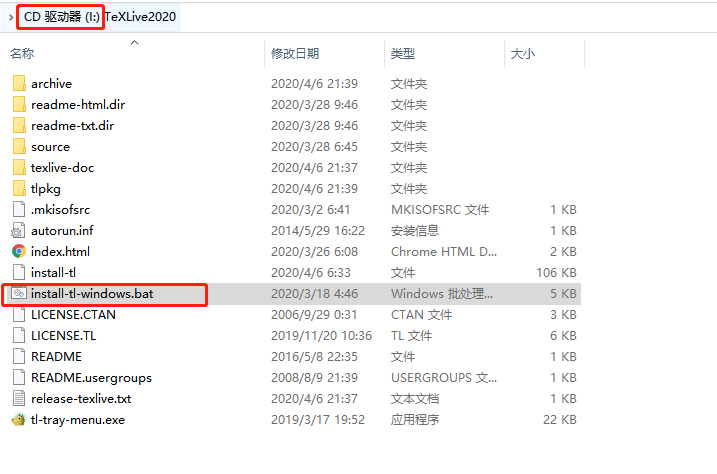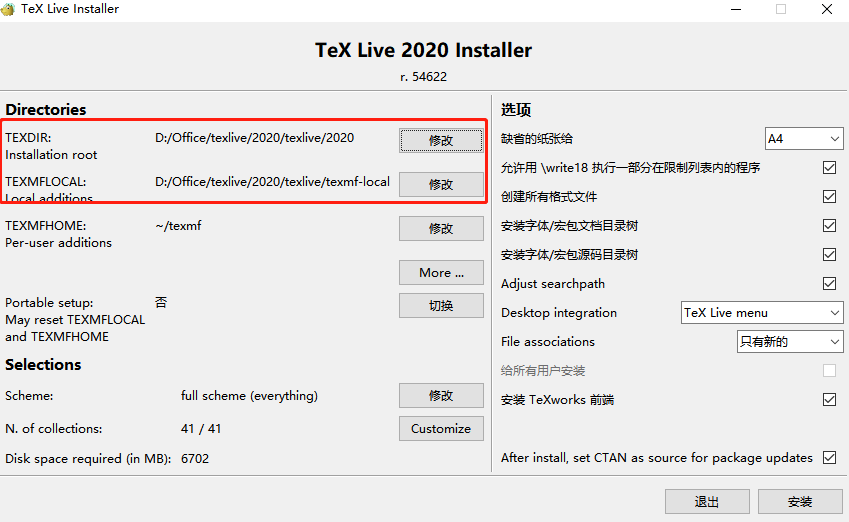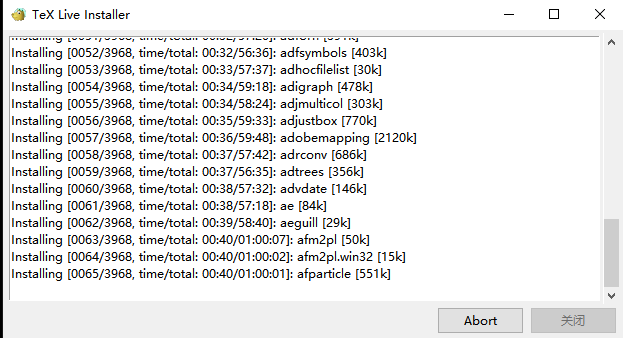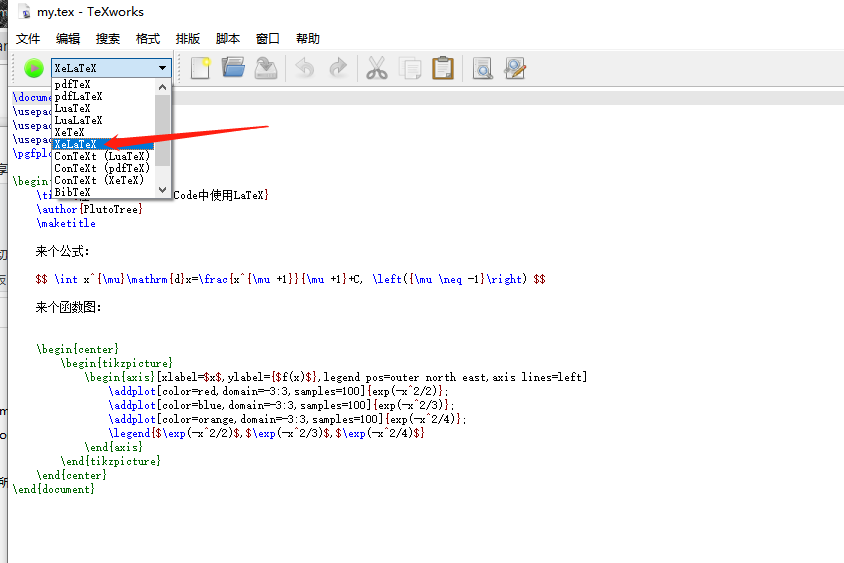## 配置Visual Studio Code

Visual Studio Code的安装就不介绍了，直接安装插件LaTeX WorkshopLaTeX Utilities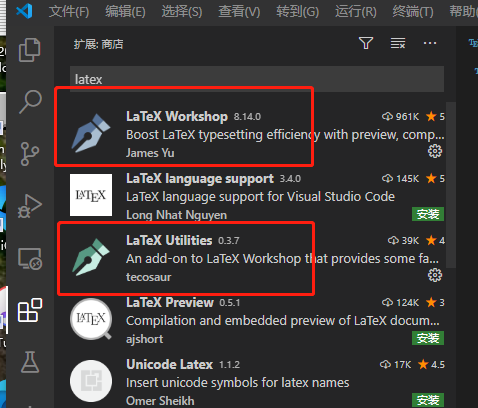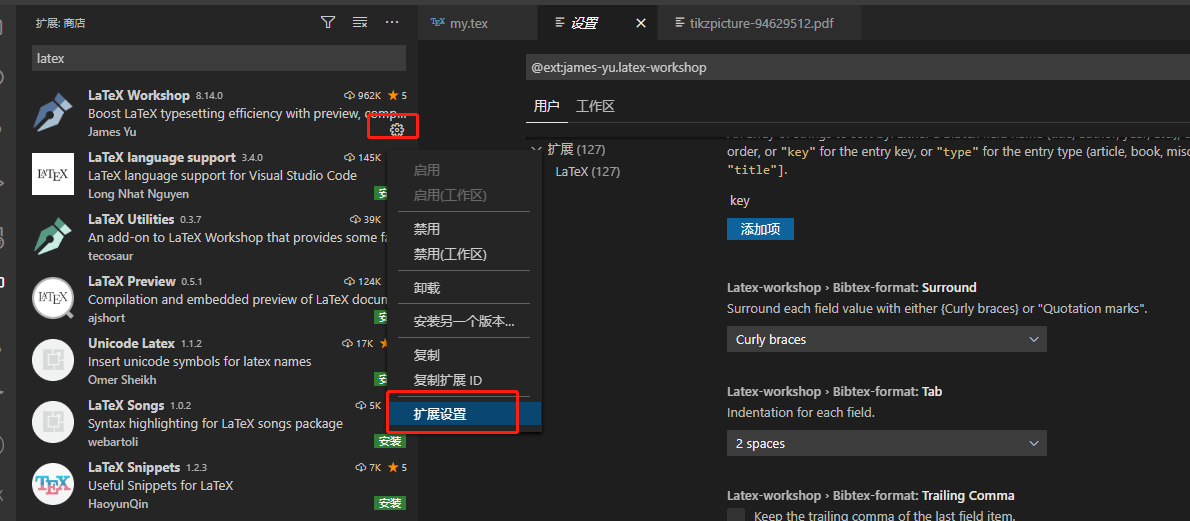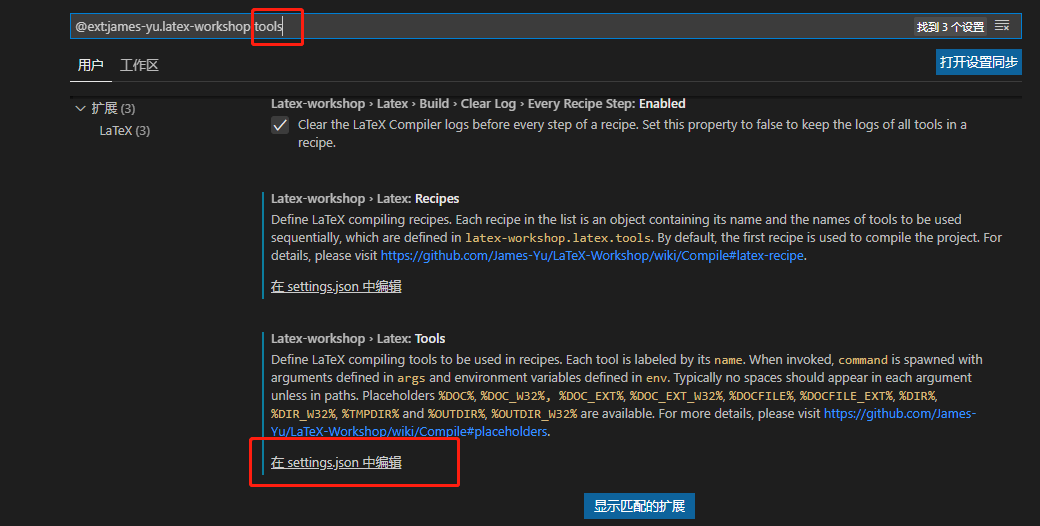{
"name": "xelatex",
"command": "xelatex",
"args": [
"-synctex=1",
"-interaction=nonstopmode",
"-file-line-error",
"-output-directory=%OUTDIR%",
"%DOCFILE%"
],
"env": {}
},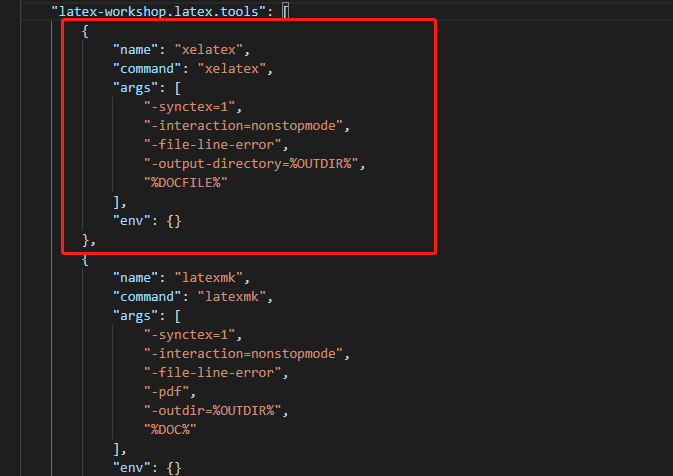{
"name": "xelatex",
"tools": [
"xelatex"
]
},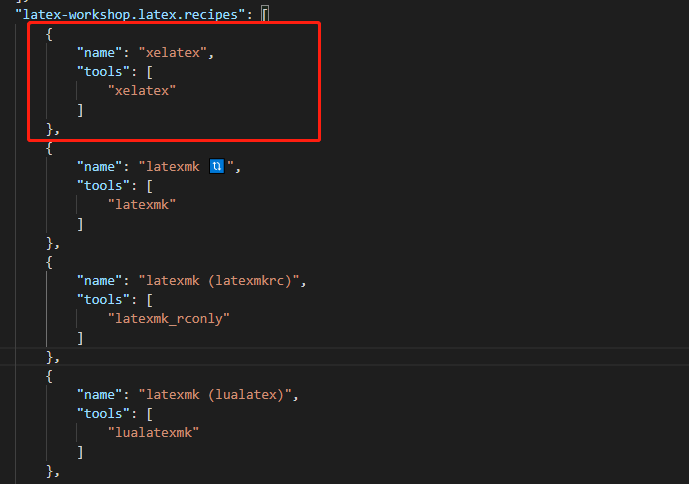## 使用说明